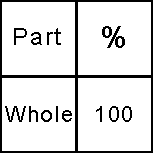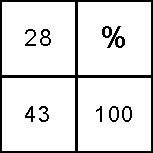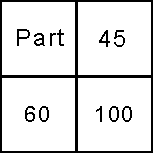# Calculate Percentage

In working through a percentage formula, there are usually three variables in play: The whole number, a part thereof and the percentage.

An easy way to organize this information is in a quadrant grid.

Percentage Calculator grid:If you know two of the variables in your equation, it is very easy to calculate the third.

1.) Take the two known diagonal quadrants and multiply them.
3.) That's it!

Find percentage:
Okay, let's say Jack has 43 beans and he sells 28 of them, what percentage did he sell?

We would set it up like this:28 X 100 = 2800
2800 divided by 43 = 65.1

The answer is 65 percent (rounded).

Find part:
Now let's give Jack 60 beans, he needs to give his mother 45 percent of them. How many beans does he need to give his mother?

The setup:60 X 45 = 2700
2700 / 100 = 27
He would give his mama 27 beans.

And that's how easy it is to calculate percentage. Finding the whole number works the same way.